# Work

Starting with this lecture, we’ll be learning about energy!

Before we talk about energy, let us first learn about the work done by forces.
Work appears in all areas of physics, not just mechanics.
If you’re fuzzy on the meaning of a term, it can hinder you in other areas, so make sure you know exactly what it means here!

## What is “work” in physics?

The word “work” is usually used in various senses, but in physics, “Work” means “Moving an object by acting a force”.
The word “work” is used in physics only in this sense, so do not put your own interpretation into it.

The amount of work can be calculated as “magnitude of force × distance traveled”, using the unit J (joule).

Since this is not enough explanation, I will explain some common calculation patterns using examples.

## 1) When force and direction of movement are the same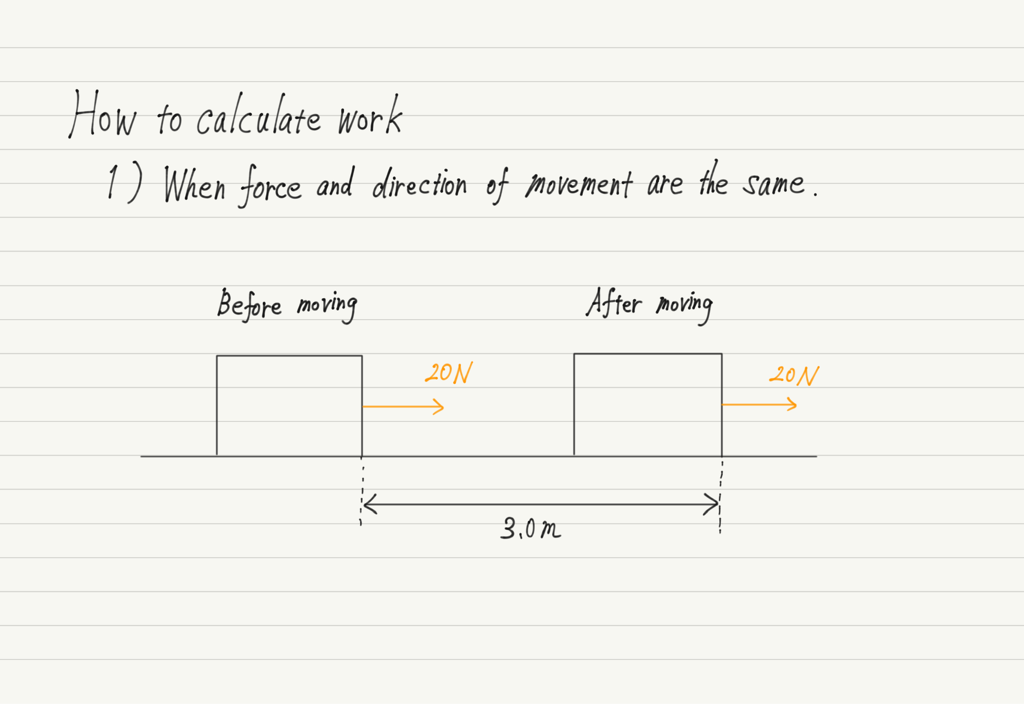If the object is moving in the right direction in response to a rightward force as shown in the figure above, the work done by this force is calculated as “magnitude of force × distance traveled”.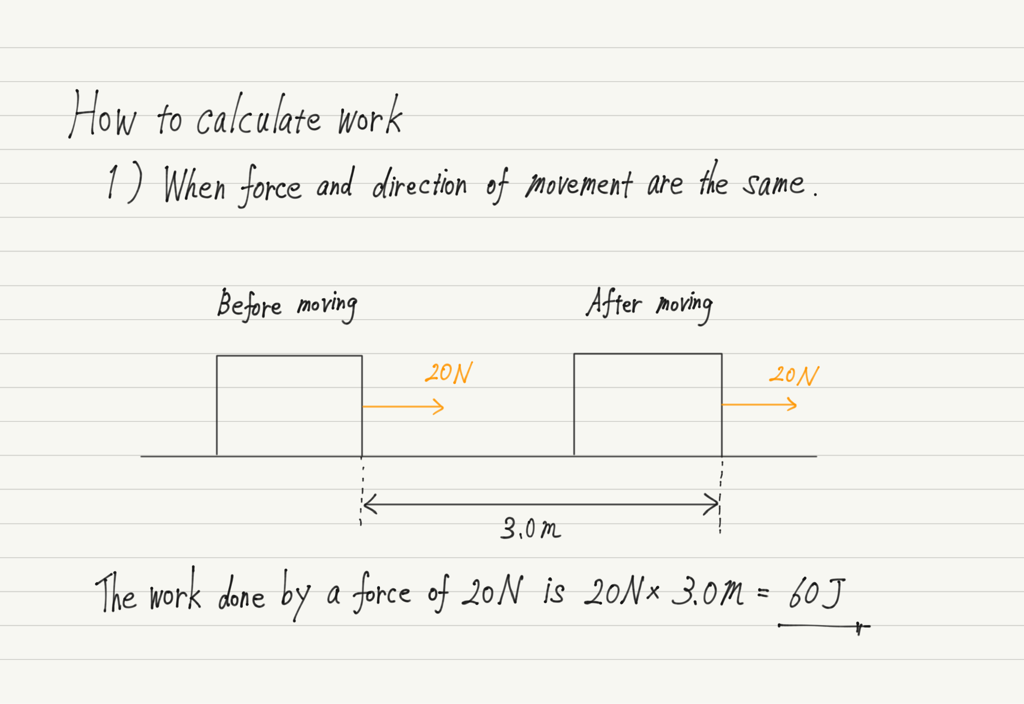This is the basis of the work calculation. It’s easy!

## 2) When force and direction of movement are opposite

It is the rightward force applied to the object in 1) that moved the object to the right.
When we hear the phrase “work done by force”, we tend to think that it means “work done by force that moves an object”, but in fact, work can also be calculated by forces other than force that moves an object.

For example, suppose that in 1) there is friction between the floor.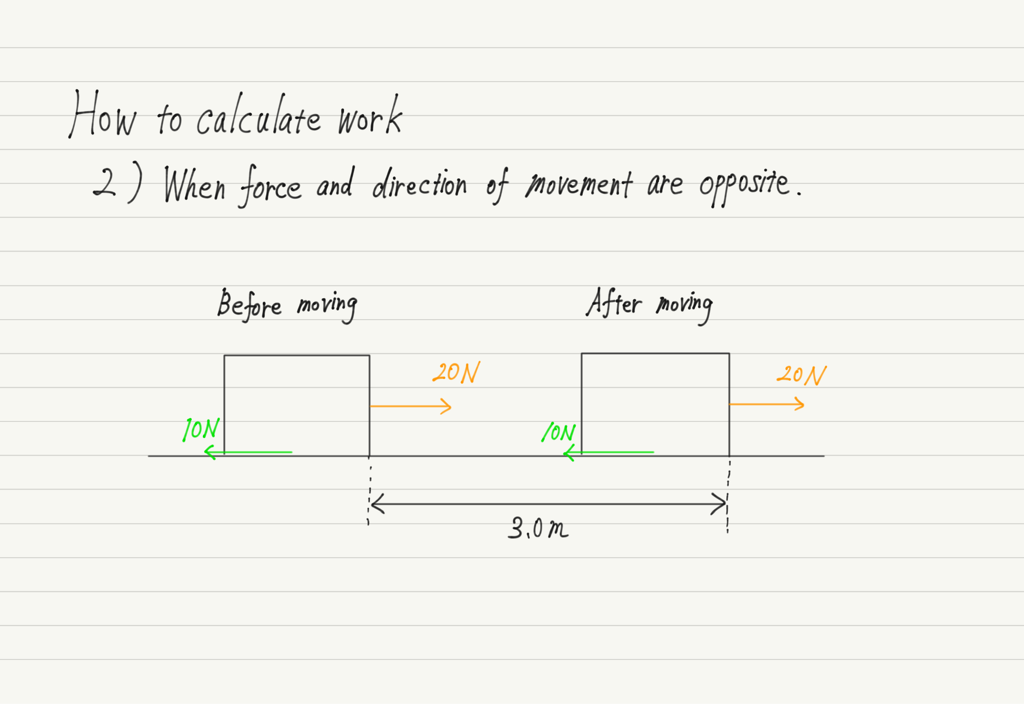This kinetic friction is not moving the object, but rather hindering its motion, but the work can be calculated.

The formula is still “force × distance traveled”, but in the case of a force in the opposite direction of the object’s movement, add a minus sign to the answer obtained in the calculation.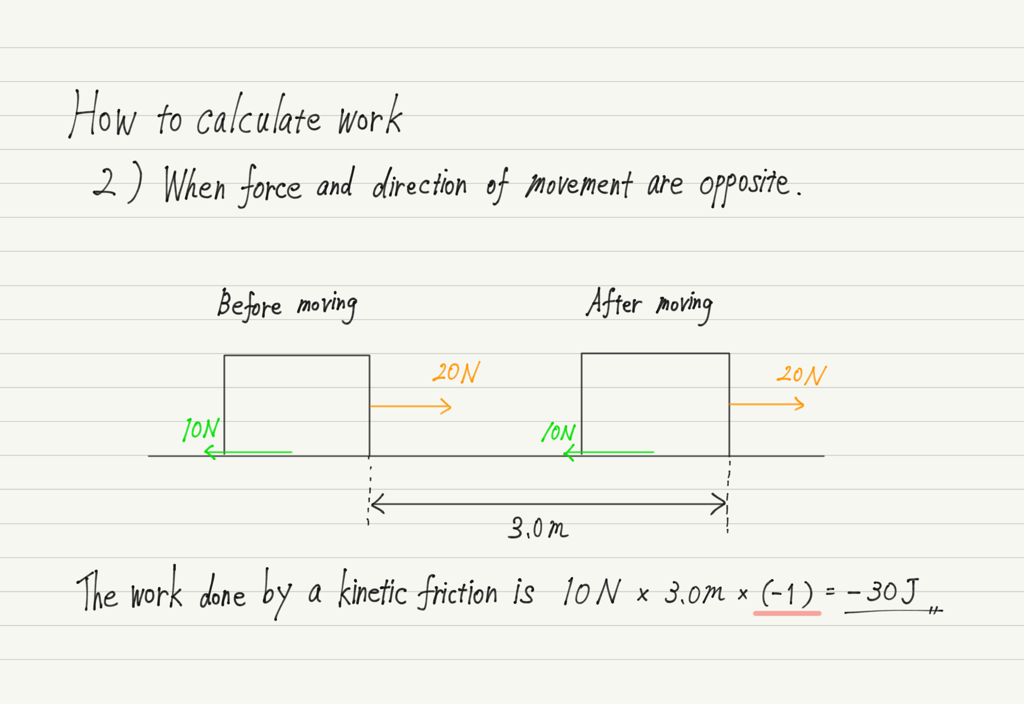There are two types of work: positive work and negative work.
Think of it as positive work if the force contributes to moving the object, and negative work if it interferes with the object’s motion.

## 3) When force and direction of movement are perpendicular

Finally, there is the case where the force and the direction of movement are perpendicular.
In this example, the gravitational force or normal force is perpendicular to the direction of motion.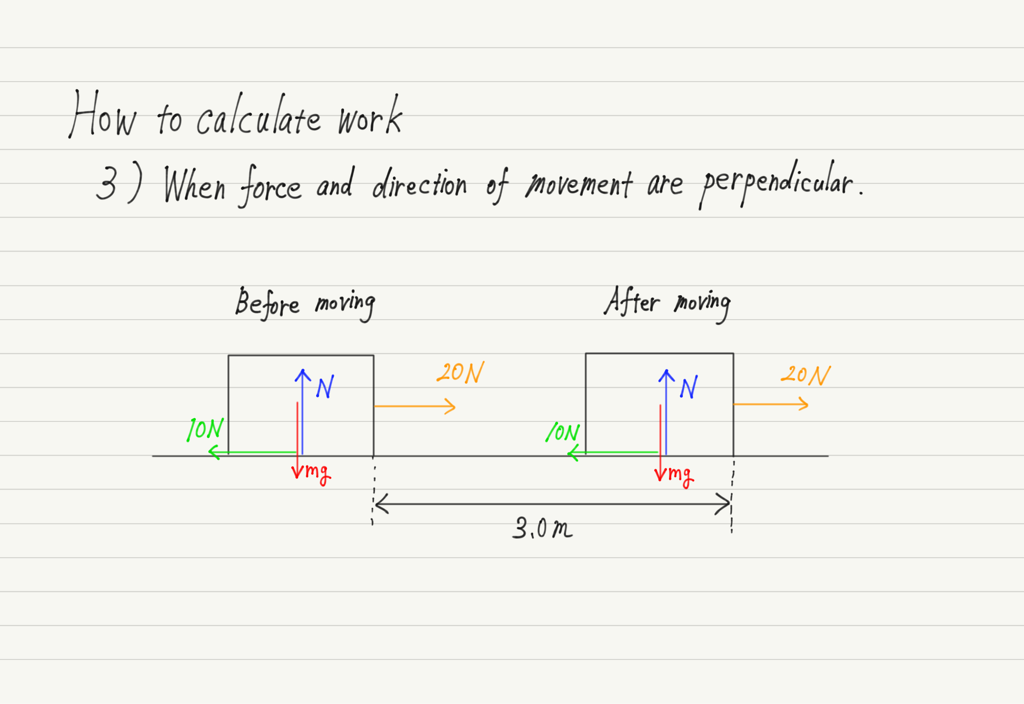In fact, the work in this case is the easiest to find.
Because the work done by a force perpendicular to the direction of motion is always zero!

In section 2), I wrote that if a force contributes to the motion of an object, it is positive work, and if it interferes it, it is negative work.
However, a vertical force neither contributes to nor interferes the motion of an object, right? That is why it is zero.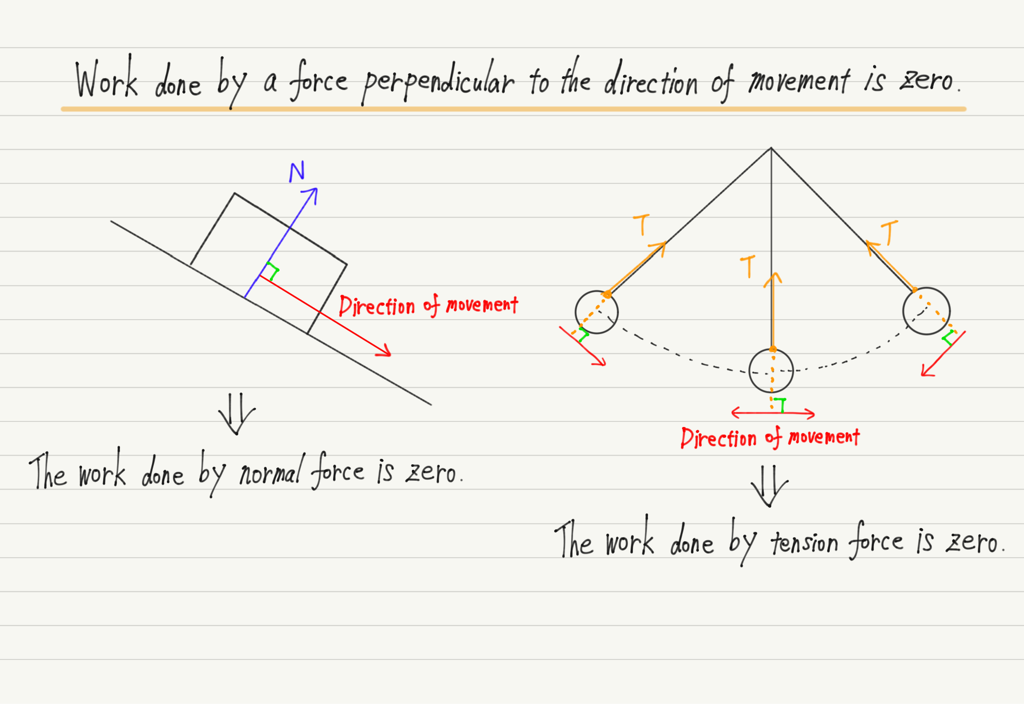## Note on work calculation

A few notes on work calculations.

First. Even if a force is applied to an object, if the object does not move, the work is zero (work = force × distance traveled, so if the distance traveled is zero, the work is also zero).

In everyday life, we often say “I did my best at work”, but in the physical world, no matter how hard you try, if an object does not move, you have not done work!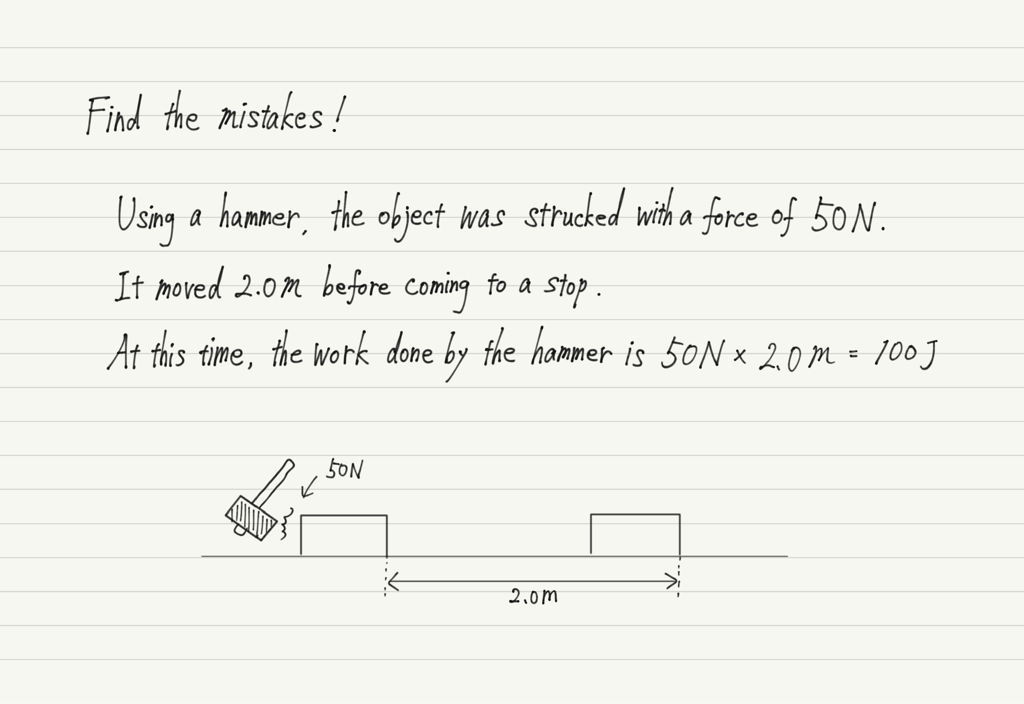At the beginning of this lecture, we said “work = moving an object by acting a force”.
However, there is one condition for calculating work as “force × distance traveled”.
It is the force that is acted to the object the entire time it is moving.

In the figure above, a force is acted to move the object, but the force is only acted for the first instant.
In such a case, multiplying the force by the distance traveled does not calculate work!

Third. The distinction between vectors and scalars is important in physics, but work is scalars, not vectors!

If you are not familiar with vectors and scalars, read the explanation from the link below.

https://www.yukimura-physics.com/entry/guide08

The fact that the work is scalar means that there is no orientation.
Negative work was found in 2), but this negative does not represent “inverse direction” but “less than zero”.
In other words, it is wrong to interpret the answer to 2) as “30J to the left”!

Because work is explained together with talk of direction of movement and direction of force, many people mistakenly believe that work also has an orientation. Be careful!

## Summary of this lecture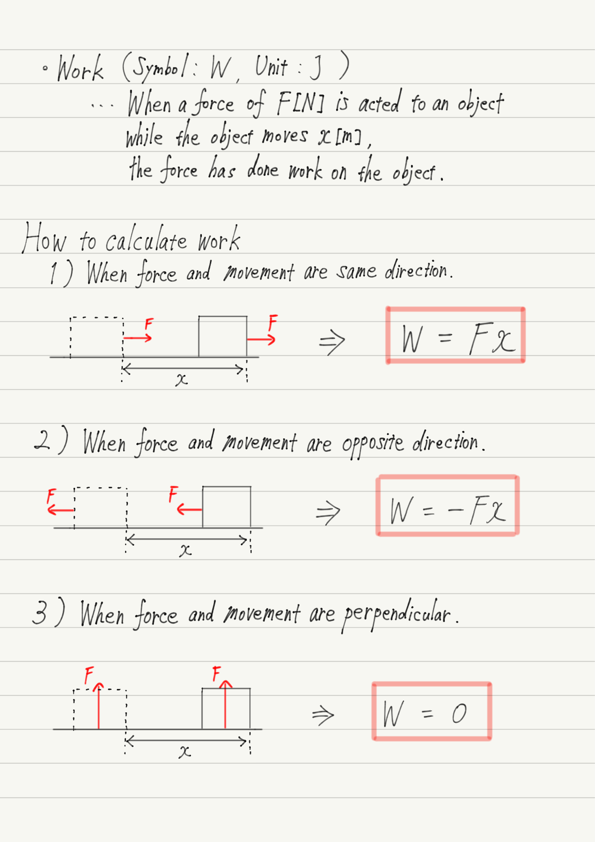## Next Time

In the next lecture, we will consider the benefits of working with tools.

https://www.yukimura-physics.com/entry/dyn-f24

error: Content is protected !!